o
osc_wws45aot

## 一、打开notebook界面的方法：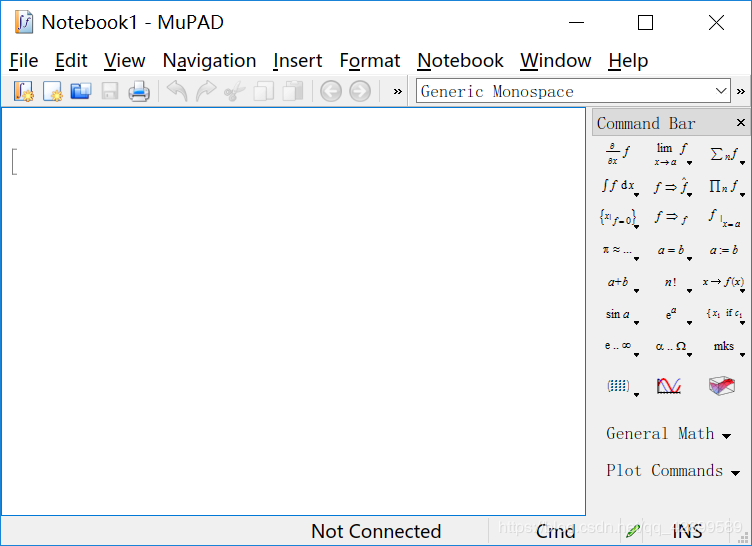## 二、notebook界面的三种区域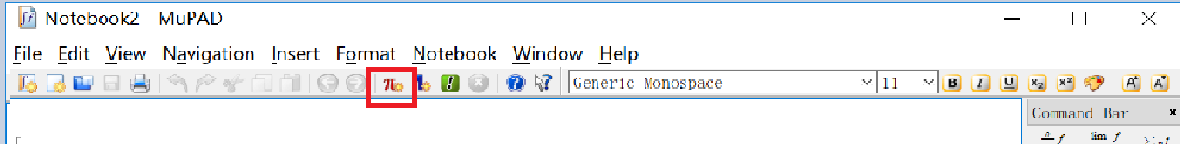（二）、输出区域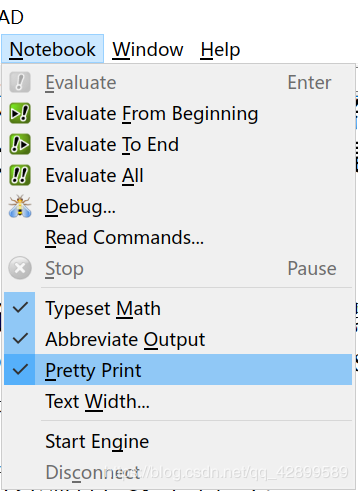``````delete x:
solve(x^3 + x^2 + 1 = 0, x, MaxDegree = 3);
``````

1.用Typeset Math和Abbreviate Output两种格式运行此代码
2.只选Typeset Math格式运行此代码：是很好看的印刷体格式，但复制是以图片格式来复制的。
3.只选Abbreviate Output格式运行：和键盘输入的数学表达式相同。
4.只选Pretty print格式：matlab符号数学工具箱原有的一种数学公式显示格式。
5.Text Width是设置输出区域每一行最大字符数。

（三）、文本区域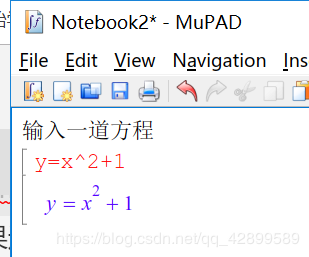## 三、基础语法

（一）、标识符

1.第一个字符不能是数字；
2.区分大小写;
3.不能是关键字;

（二）、赋值

<标识符>：=value
value可以是数、表达式、方程、列表、集合、矩阵等。
y:=a+x;

``````a:=10;
``````

``````delete a；
``````

（三）、标准库的特殊记号

``````E          自然对数e
exp(n)     e的n次幂
I          虚数单位i
PI         圆周率
infinity   正无穷大
-infinity  负无穷大
undefined  无定义
``````

``````RD_INF      浮点数形式的正无穷大
RD_NINF     浮点数形式的负无穷大
RD_NAN      浮点数形式的无定义
``````

``````TRUE       真
FALSE      假
UNKNOWN    未知
``````

``````C_         复数集
R_         实数
Q_         有理数
Z_         整数
N_         正整数
``````

``````::         连接库名和函数名
'          单引号
[]         下标运算符
.          连结运算符，小数点
@@         函数复合迭代运算符
@          函数符合运算符
!          阶乘运算符
!!         双阶乘
^          乘幂
*          乘法
/          除法
-          负号或减
+          加
..         范围（区间）运算符
in         属于（集合）
=
<>         不相等
>;<;<=;>=
\$          生成序列
and        与
not        非
xor        异或
or         或
assuming   假设（临时性的）
,          逗号，分隔序列用的
;          命令结束，显示结果
:          命令结束，不显示结果
``````

``````sin cos tan cot sec csc
arcsin arccos arctan arccot arcsec arccsc
sinh cosh tanh coth sech csch
arcsinh arccosh arctanh arccoth arcsech arccsch
exp  ln log log2 log10 abs(绝对值，复数模)
binomial   二项展开式系数
gamma      伽马函数
max        最大值
min        最小值
sign       符号函数
sqrt       算术平方根
surd       n次方根
ceil       上取整（2.5->3）
floor      下取整(2.5->2)
round	   四舍五入
trunc 	   截去小数部分取整
Re         复数的实部
Im         复数的虚部
arg        复数的幅角主值
delete     清除标识符的值
domtype    查看数据类型
eval       计算符号值
float      计算浮点数近似值
%          最近的计算结果
last(n)或%n 最近的第n个计算结果
plot       显示图形对象
``````

（四）、数据类型

``````DOM_INT			整数
DOM_RAT 		有理数
DOM_COMPLEX		复数
DOM_FLOAT	    浮点数
DOM_BOOL		逻辑常数
DOM_IDENT		标识符 *
DOM_EXPR		表达式 *
DOM_POLY		多项式
DOM_STRING		字符串
DOM_LIST		列表 *
DOM_SET			集合 *
DOM_ARRAY		数组
DOM_TABLE		列表
DOM_PROC		过程
matrix			矩阵和向量
ode				常微分方程
piecewise		条件定义对象
Series::Puiseux		截断式级数展开式
O			级数展开式的大O项
rec			递推方程，差分方程
``````

（五）、命令语句
1.处理表达式
(1).subs 代入

``````subs(a+b+c,a=x^2,b=10);
``````

(2).subsex 比subs的范围更大

``````subsex(a+b+c,a+b=x^2);
``````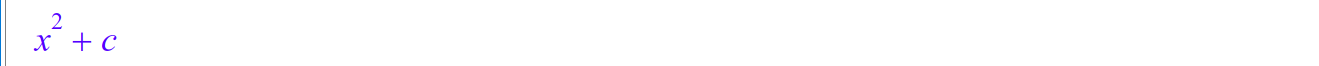(3).simplify 化简命令

simplify(f)

``````simplify(exp(2*(3/2))-exp(3))
``````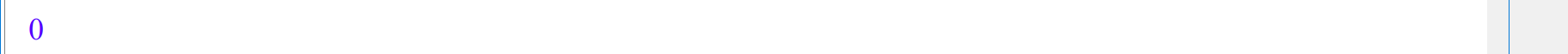``````f:=(cos(x)^2-sin(x)^2)/(sin(x)*cos(x));
simplify(f);
Simplify(f);
``````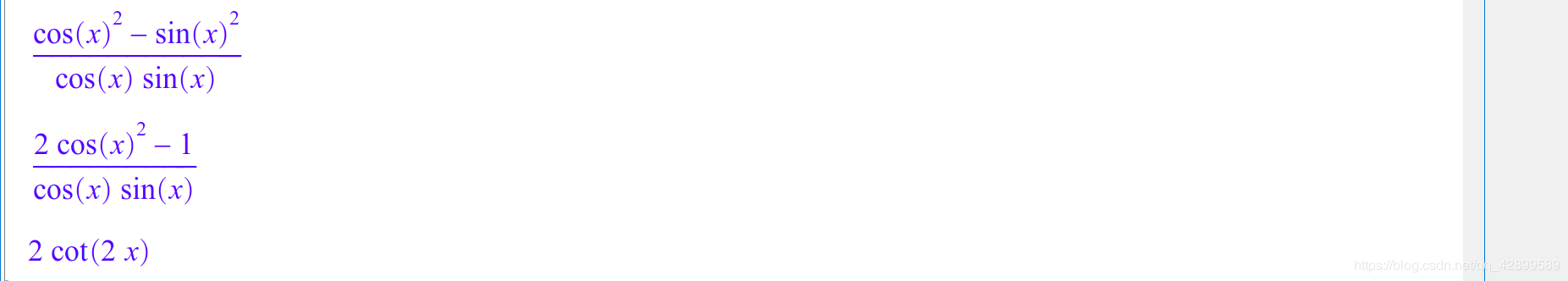(4).combine 合并

``````combine(sqrt(2)*sqrt(5))
``````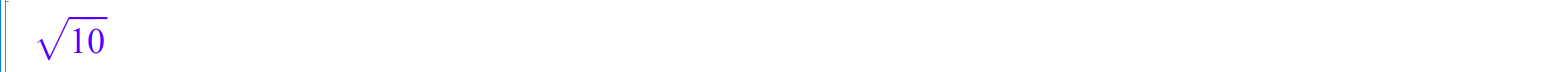(5).collect 合并

collect(f,[x,y,z,…])

``````f:=x^2*y+z*x*y*y;
collect(f,x);
``````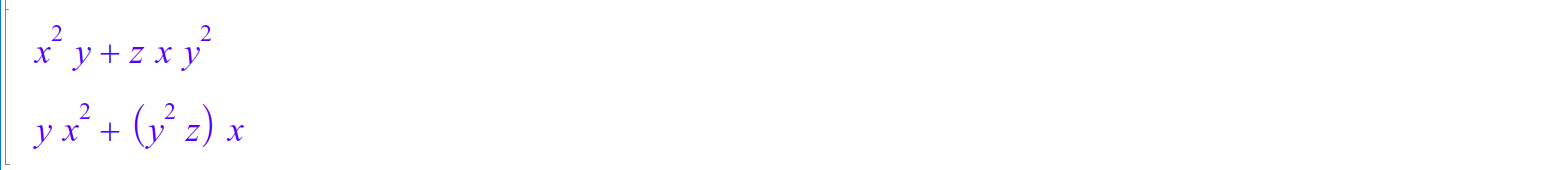``````f:=x^2*y+z*x*y*y+z*y*y*x+x*x*y*z;
collect(f,[x,y]);
``````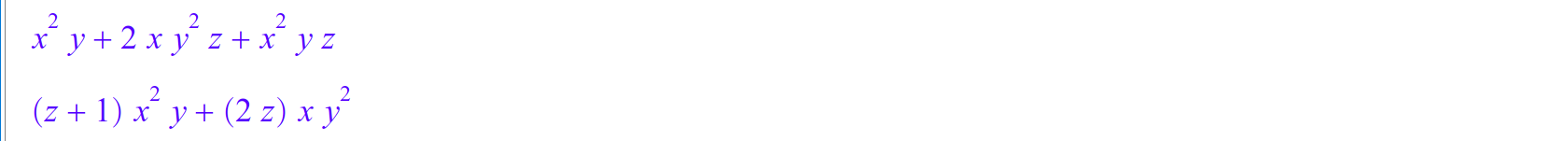(6).expand 展开

expand(f)

``````expand((z+1)*x*x*y+(2*z)*x*y*y)
``````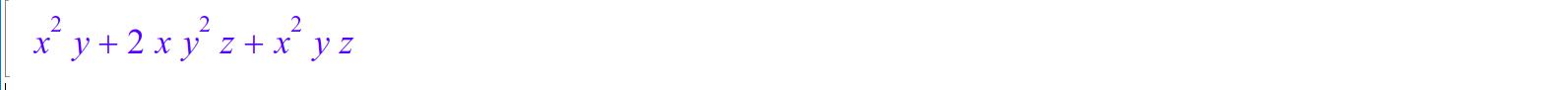(7).factor 分解因式

factor(f)

``````factor(x^2-3*x+2)
``````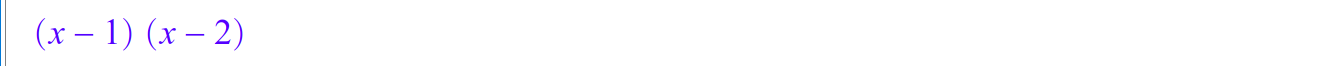(8).normal 和simplifyFraction 化简分式

normal(f)
simplifyFraction(f)

``````p:=x/(x^6-1)+x^2/(x^4-1);
normal(p)
``````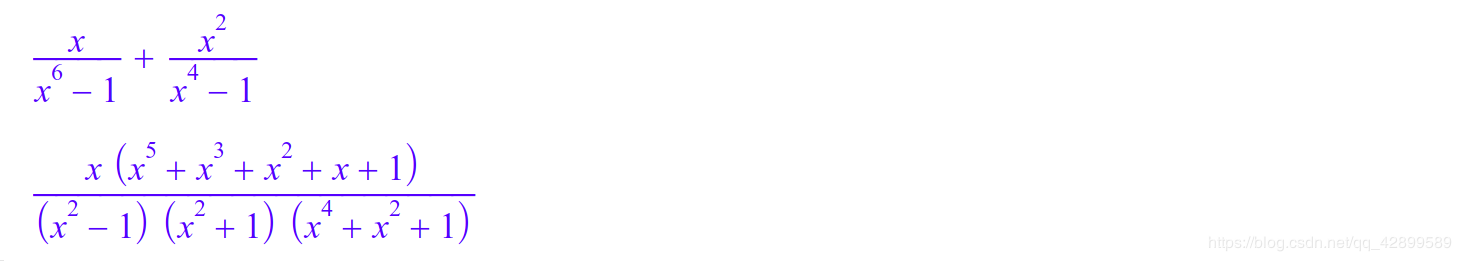``````r:=sqrt(3*sqrt(3+2*sqrt(5-12*sqrt(3-2*sqrt(2))))+14);
``````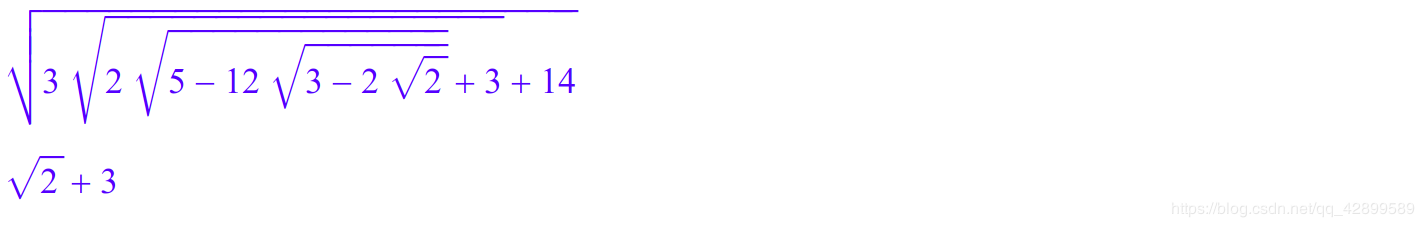2.列表

``````[1,2,3,4,5]
``````

``````[\$1..5]
``````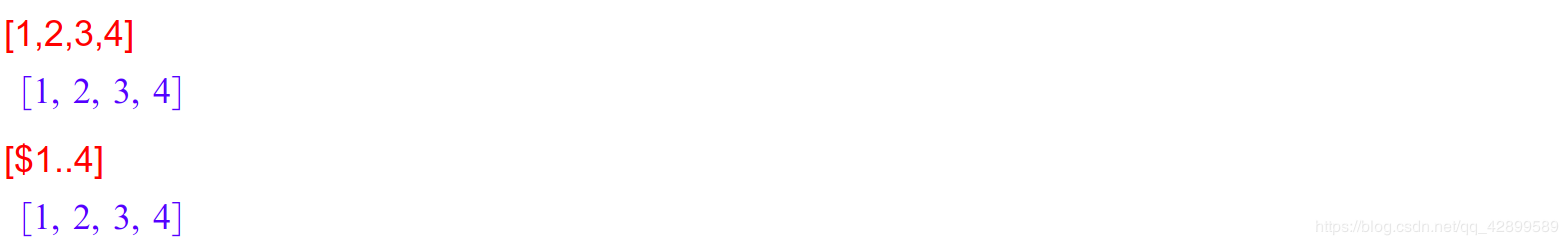``````[x_.i \$i=1..9 step 2]
``````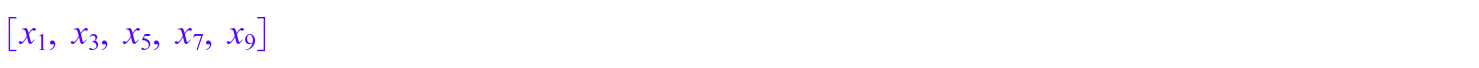x与i之间用下划线_与.连结运算符(句点)连接, 注意step后的空格不能少

``````\$a..b				a,a+1,a+2,...,b
\$a..b step c		a,a+c,a+2c,...,b
f\$n 				重复n个f组成的序列
f(i)\$i=a..b			f(a),f(a+1),f(a+2),...f(b)
f(i)\$i=a..b step c
f(i)\$i in list		 list是列表
f(i)\$i in set  		 set是集合
``````

3.集合

``````?collect
``````

## 四、函数的创建

（一）、用赋值运算符创建函数

``````y:=2*x-1
``````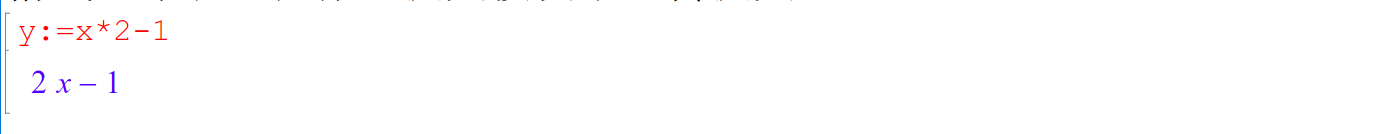(二)、用映射创建函数

f:=x–>body 简化函数后再创建函数
f:=x->body 直接创建函数

``````f:=x-->x*2-1;
``````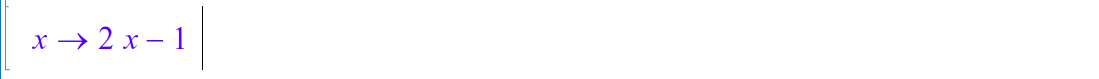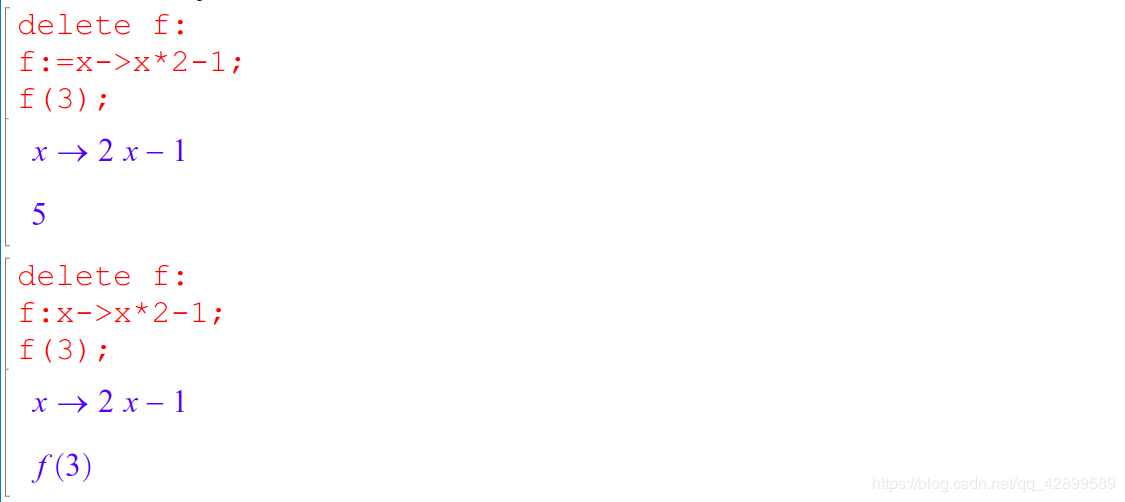（三）、创建分段函数
1.piecewise

piecewise([条件1，对象1],[条件2,对象2],…)

``````f:=x-->piecewise([x>0 or x<-1,x],[x>=-1 and x<=0,-x]);
``````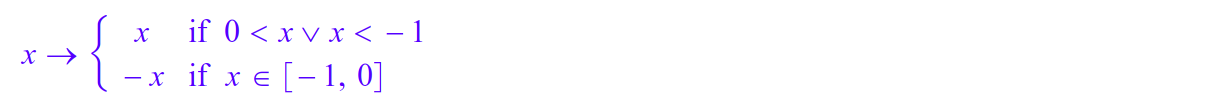``````pw:=x->piecewise([x>0 and x < 1,1],[Otherwise,0]);
//Otherwise代表不包含在已有condition中的其他情况：
``````

(四)、复合函数的创建

f@g

``````f:=x-->piecewise([x<1 or x>-1,1],[x=1 or x=-1,0],[x>1 or x<-1,-1]);
g:=x->exp(x);
f@g;
``````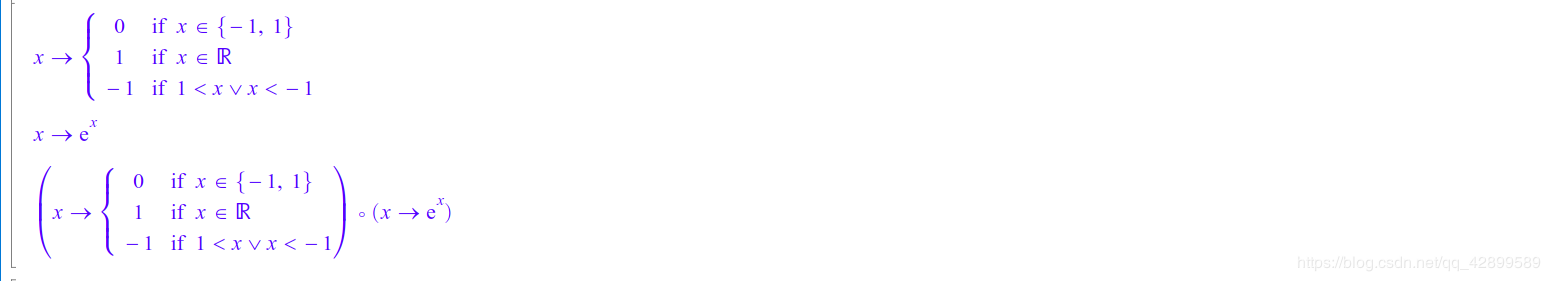(五)、调用系统函数

``````y:=x->sin(x);
//注意括号不能省，否则系统会把sinx当成一个标识符，如图
``````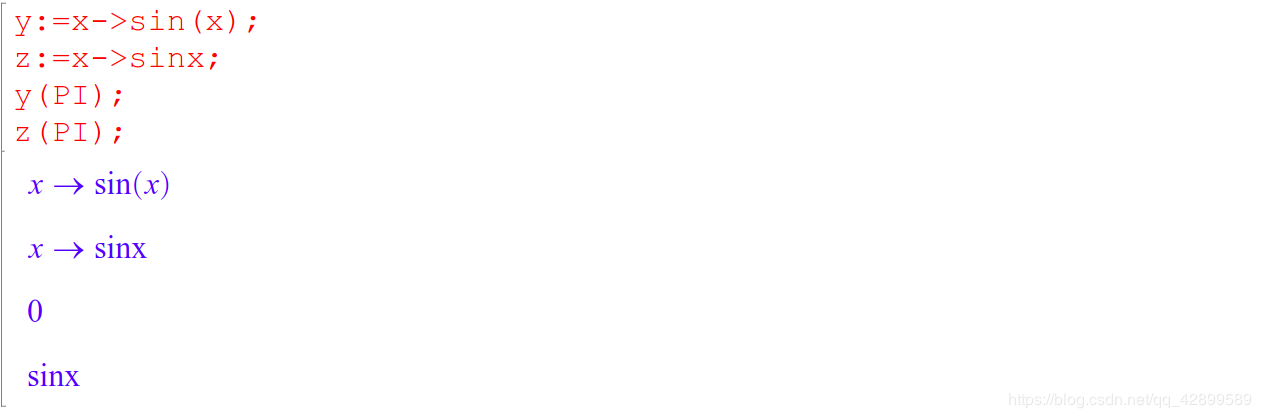``````g:=(x,y)->x^2+y^3
``````

## 五、显示函数图形

(一)、plot(目标，<可选项>，<属性>)

``````图形属性								简写	功能						默认
Scaling=Constrained					#C		所有坐标轴单位长度相等	自动设置
GridVisible=TURE					#G		显示坐标网格				无网格
Axes=Origin						    #O		坐标系包含原点			自动设置
AxesVisible=FALSE					无		不显示坐标轴				显示坐标轴
ViewingBox=[x1..x2,y1..y2] 			无		二维坐标轴范围			自动设置
ViewingBox=[x1..x2,y1..y2,z1..z2]	无		三维坐标轴范围			自动设置
Color=[r,g,b] 或 RGB::name			无		图形颜色					略
Height=m							无		图形高度					80
Width=n								无		图形宽度					120
Footer=text 						无		在图像底部添加文本（字符串）无

LineStyle=Solid			实线
LineStyle=Dashed		虚线
LineStyle=Dotted		点线
LineWidth=n				线宽，默认为0.35
Mesh					采样点数目（与曲线的光滑程度有关，越大越光滑），默认为Mesh=121
Submesh					额外采样点的密度，默认Submesh=0

PointStyle				点形状，默认值是PointStyle=FilledCircles
8种：Squares, FilledSquares, Circles, Crosses, XCrosses, Diamonds, FilledDiamonds, Stars
PointSize				点尺寸，默认值是PointSize=1.5，单位是毫米.
``````

RGB色彩模式

``````Color=RGB::Red		Color=[1,0,0]
Color=RGB::Green	Color=[0,1,0]
Color=RGB::Blue		Color=[0,0,1]
``````

（二）、创建显函数的二维图像
1.plot::Function2d(f,x=xmin…xmax,<动画参数>，<属性>)

``````f:=x->sin(x);
g:=plot::Function2d(f,x=0..PI);//此时仅仅是创建了一个图形赋值给g
plot(g);//生成图形
``````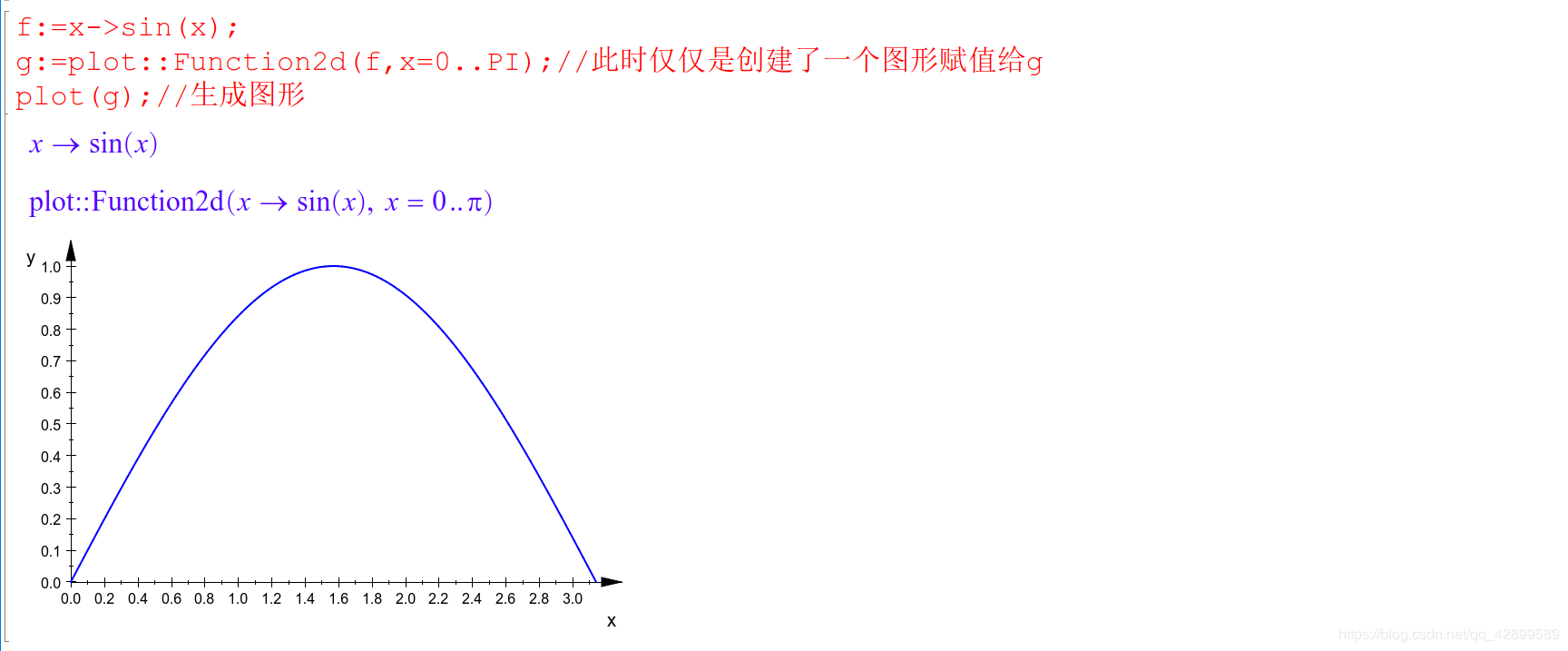``````g:=plot::Function2d(f,x=0..PI,Color=RGB::Red,LineStyle=Dashed);
plot(g,#C,#G)
``````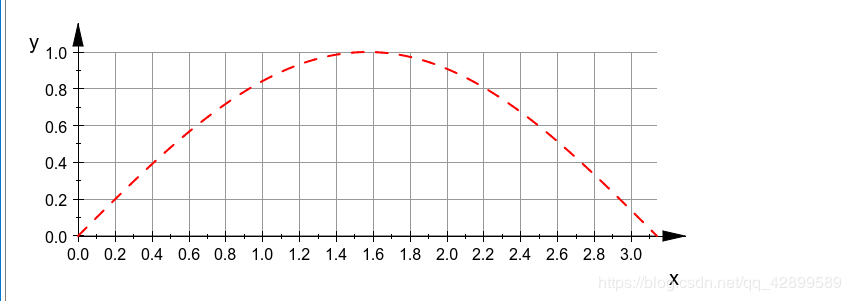``````g:=plot::Function2d(sin(x),x=0..2*PI,Color=RGB::Red,LineStyle=Dashed,Mesh=4,AdaptiveMesh=0);
plot(g,#C,#G)
``````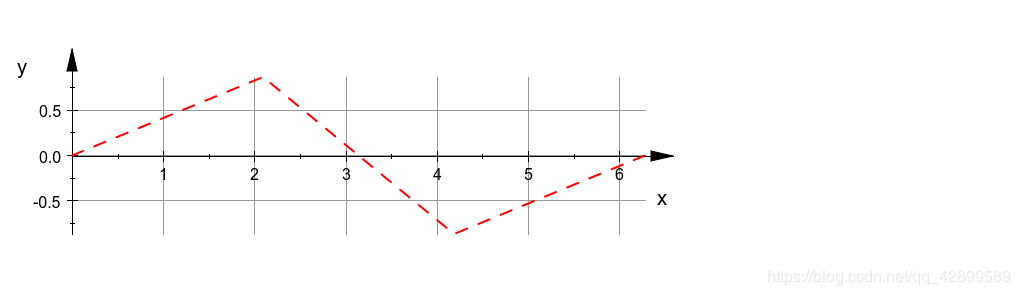2.plot::Curve2d([x,y],t=tmin…tmax,<动画参数>,<属性>)

``````f:=plot::Curve2d([sin(t),cos(t)]);
plot(f,#C);
``````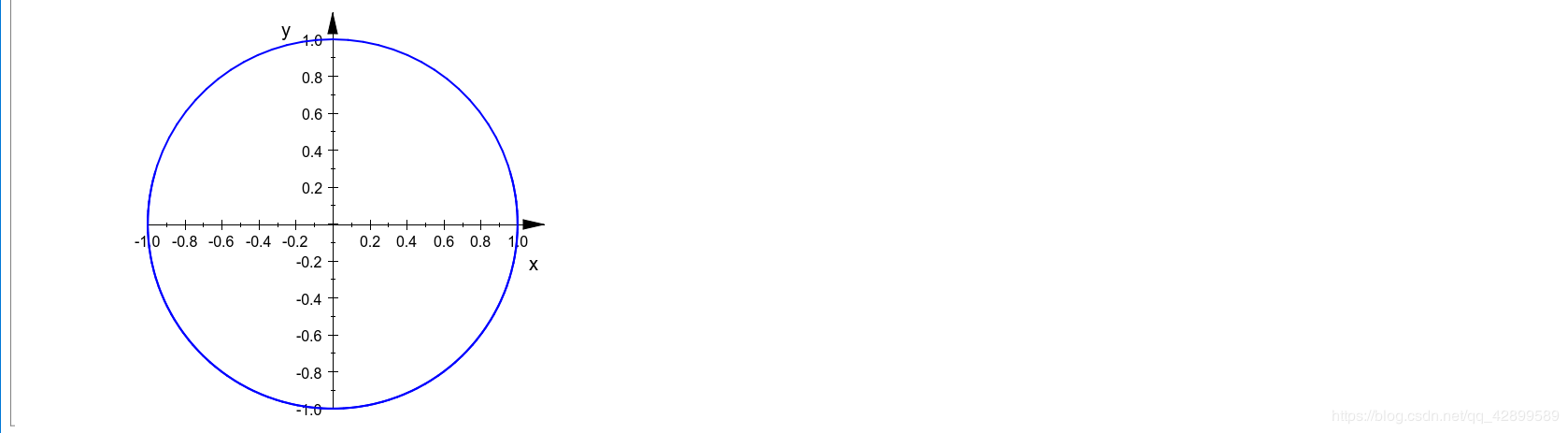3.plot::Implicit2d(f,x=xmin…xmax,y=ymin…ymax,<动画参数>,<属性>)

``````plot(plot::Implicit2d((x-y)*(x+y),x=-3..3,y=-3..3))
``````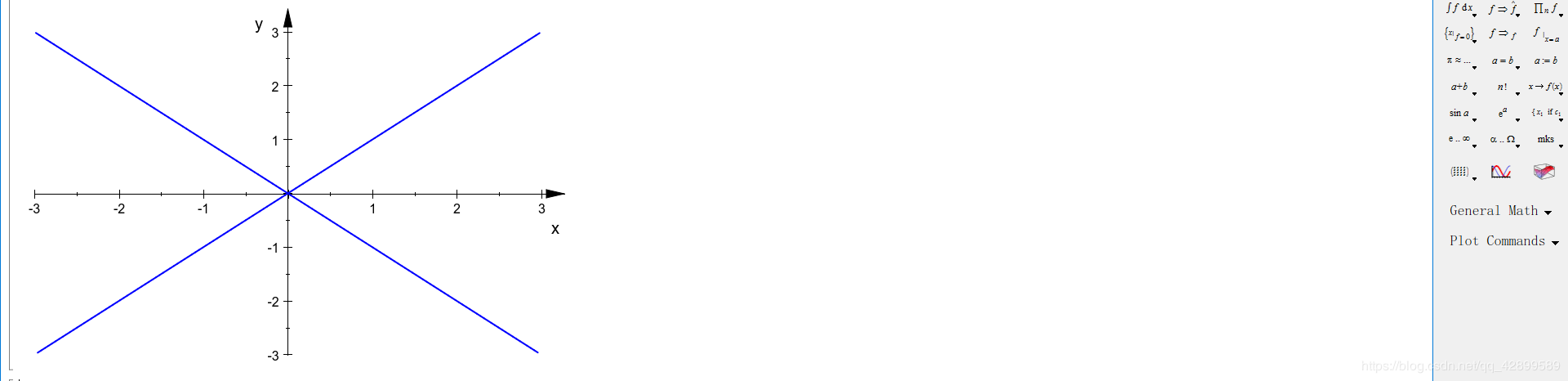4.plot::Polar([r,u],v=vmin…vmax,<动画参数>,<属性>)

``````plot(plot::Polar([2*(1-cos(r)),r],r=0..2*PI))//绘制心形曲线
``````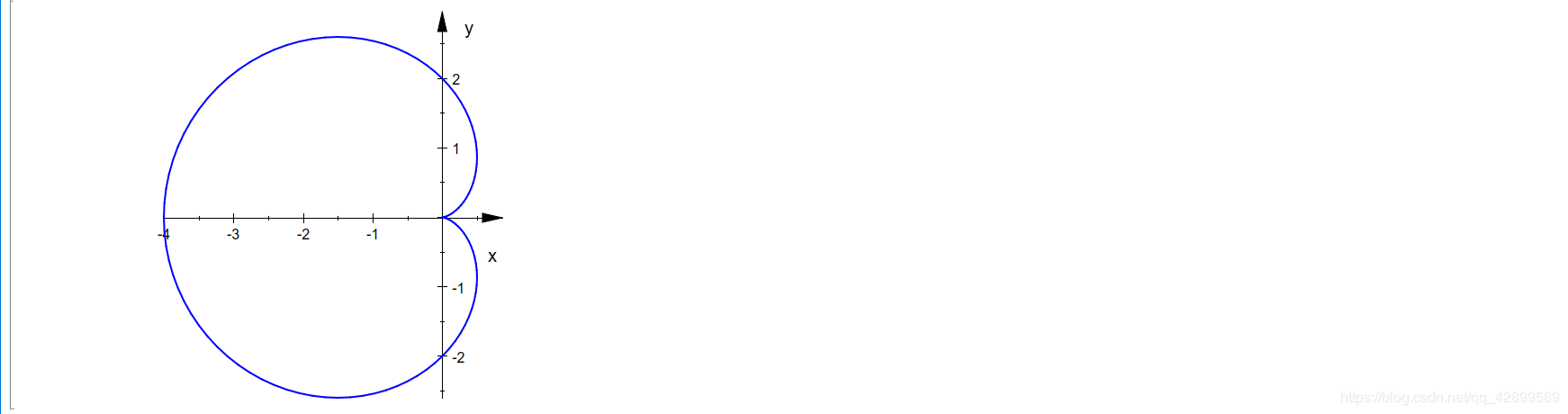``````//比较以下两个代码的区别
g:=plot::Polar([2*(1-cos(r)),r],r=0..2*PI,Mesh=13);
plot(g);//只有这个代码修改的Mesh值有效

g:=plot::Polar([2*(1-cos(r)),r],r=0..2*PI);
plot(g,Mesh=13);
``````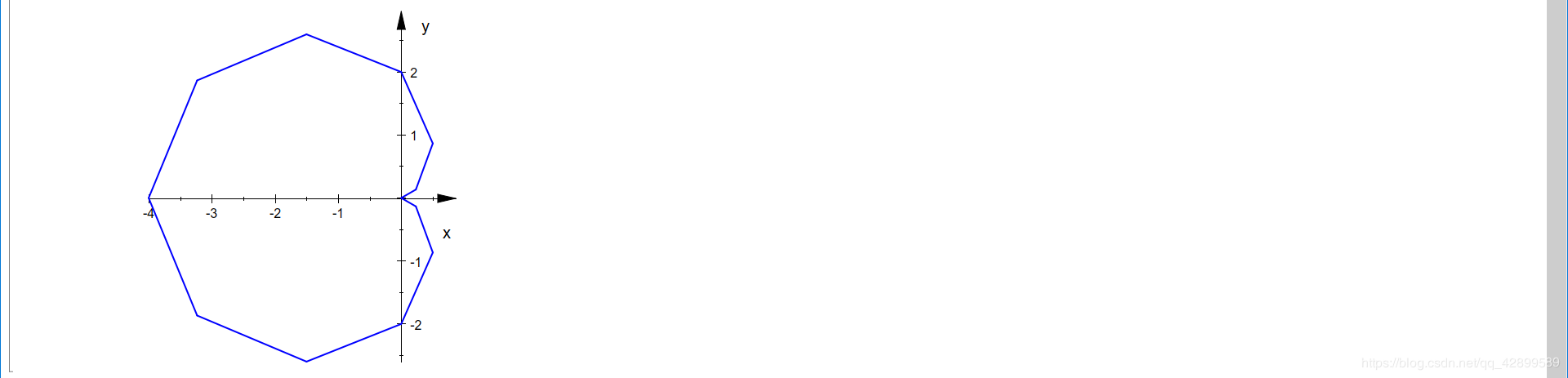（三）、绘制点、点列和直线段
1.绘制点
plot::Point2d(x,y,<动画参数>,<属性>)
plot::Point2d([x,y],<动画参数>,<属性>)
plot::Point2d(matrix[x,y],<动画参数>,<属性>)

PointStyle 点形状，默认值是PointStyle=FilledCircles
8种：Squares, FilledSquares, Circles, Crosses, XCrosses, Diamonds, FilledDiamonds, Stars
PointSize 点尺寸，默认值是PointSize=1.5，单位是毫米.

``````A:=plot::Point2d(0.5,0.5,PointStyle=Squares);
B:=plot::Point2d(0,0,PointSize=5);
plot(A,B,Width=50,Height=50)
``````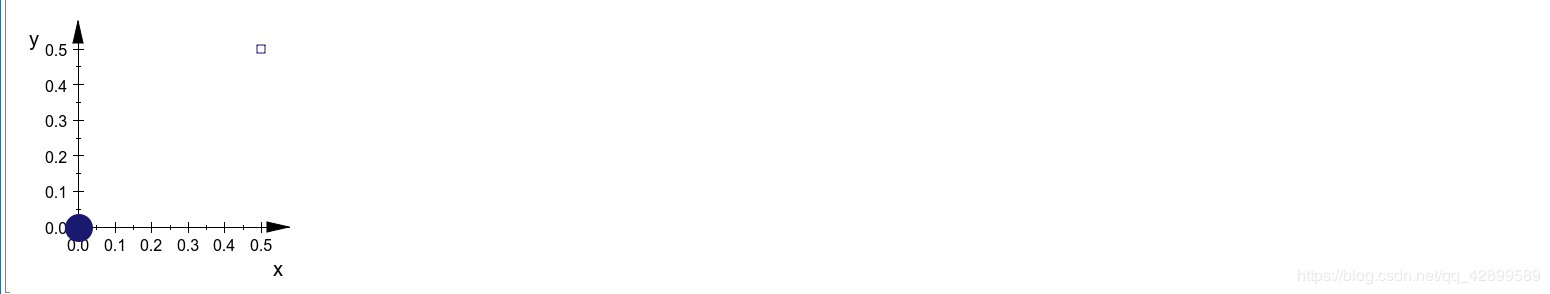2.绘制点列
plot::PointList2d(pts,<>,<>)
plot::PointList2d(M,<>,<>)

pts :点坐标的列表，即点列 [[x1,y1],[x2,y2],[x3,y3],…]

M:点坐标的n*2矩阵，如matrix([[x1,y1],[x2,y2],…])

``````Alist:=plot::PointList2d([[1,1],[2,2],[3,3]]);
plot(Alist);
//或者用序列 注意\$前没有逗号
Alist:=plot::PointList2d([[i,i]\$i=1..3]);
plot(Alist)
``````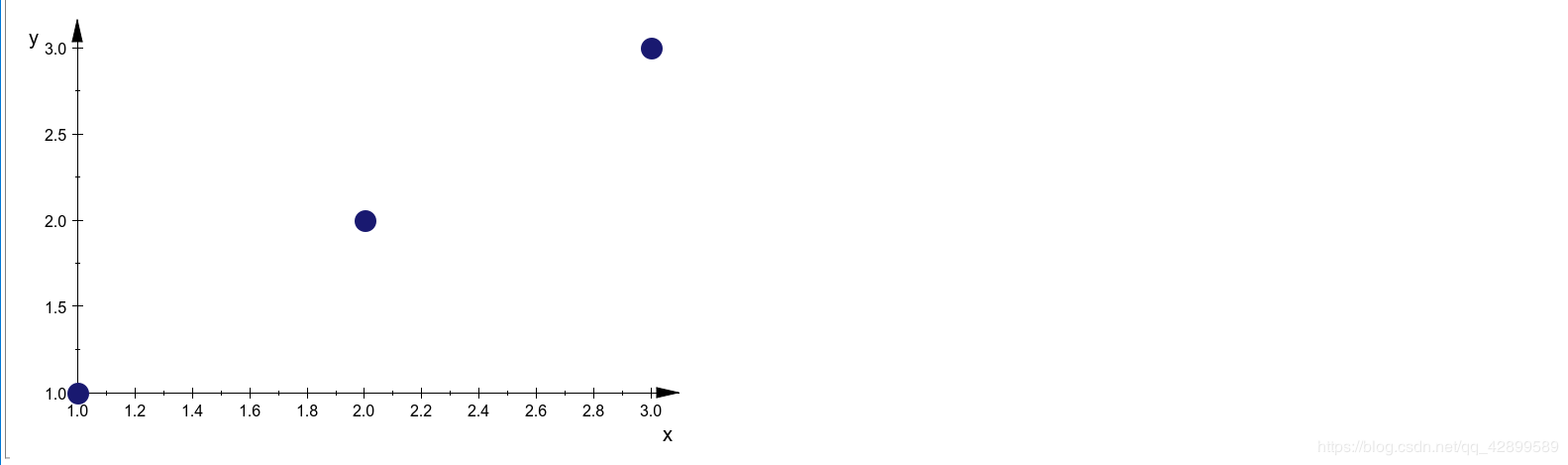3.绘制直线段
plot::Line2d([x1,y1],[x2,y2],<动画参数>,<属性>)

``````L:=plot::Line2d([1,1],[2,2])
plot(L)
``````

4.添加文本
plot::Text2d(“text”,[x,y],<动画参数>,<属性>)
“text”:要添加的文本，必须用双引号括住
[x,y] :要添加文本的位置

``````TextFont    		文本的字体，默认值是["sans-serif", 11]；
TextRotation		文本的旋转角度，弧度制，默认值是0；
HorizontalAlignment	文本相对于添加位置的水平方位，默认值是Left，还有Center和Right
VerticalAlignment	文本相对于添加位置的垂直位置，默认值是BaseLine，还有Bottom、Center和Top
``````

``````T:=plot::Text2d("haa",[1,2*a],a=0.1..2,TextFont=);
R:=plot::Rectangle(0..2,0..4);
plot(T,R)
``````
``````
T1:=plot::Text2d("ha",[1,1],TextRotation=PI/4,HorizontalAlignment=Left,TextFont=);
T2:=plot::Text2d("haa",[1,-1],TextRotation=-PI/4,HorizontalAlignment=Left,TextFont=);
T3:=plot::Text2d("haaa",[-1,-1],TextRotation=PI/4,HorizontalAlignment=Right,TextFont=);
T4:=plot::Text2d("haaaa",[-1,1],TextRotation=-PI/4,HorizontalAlignment=Right,TextFont=);
plot(T1,T2,T3,T4,#C)
``````

## 六、绘制数列、圆、多边形图像

1.plot::Sequence(x,n=nmin…nmax,<动画参数>,<属性>)
x：即x(n),数列的通项公式
n：必须都是整数

Color=RGB::Red
LinesVisible 线是否显示，默认是LineVisible=FALSE，即不显示
PointVisible 点是否显示，默认是PointVisible=TRUE，即显示

``````delete n:
A:=n-->1/2*sin(2*PI/(6*2^(n-1)))*6*2^(n-1);
ap:=plot::Sequence(A,n=0..8);
plot(ap)
``````

2.plot::Circle2d(r,[x,y],<动画参数>,<属性>)
r:半径
[x,y]:圆心，若不写，默认在原点

LinesVisible 线是否显示，默认是LineVisible=TRUE，即显示
Filled 内部是否填充，默认是Filled=FALSE,即不显示
FillColor 内部填充颜色，默认是FillColor=RGB::Red
3.绘制多边形
plot::Polygon2d(pts,<动画参数>,<属性>)
plot::Polygon2d(M,<动画参数>,<属性>)

pts：[[x1,y1],[x2,y2],…]
M：n*2规模矩阵 matrix([[x1,y1],[x2,y2]…])

LineStyle 线是否显示，默认显示，此时可以设置关于线属性的其他参数
Closed 默认Closed=FALSE，即终点与起点不连接
FillColor 内部填充颜色
Fillpattern 填充方式
PointVisible 是否显示点

``````C:=plot::Circle2d(1,[0,0]);
n:=1;
Vie:=plot::Polygon2d([[cos(2*PI*k/(6*2^(n-1))),sin(2*PI*k/(6*2^(n-1)))]\$k=0..6*2^(n-1)]);
plot(C,Vie)//绘制圆内接正多边形
``````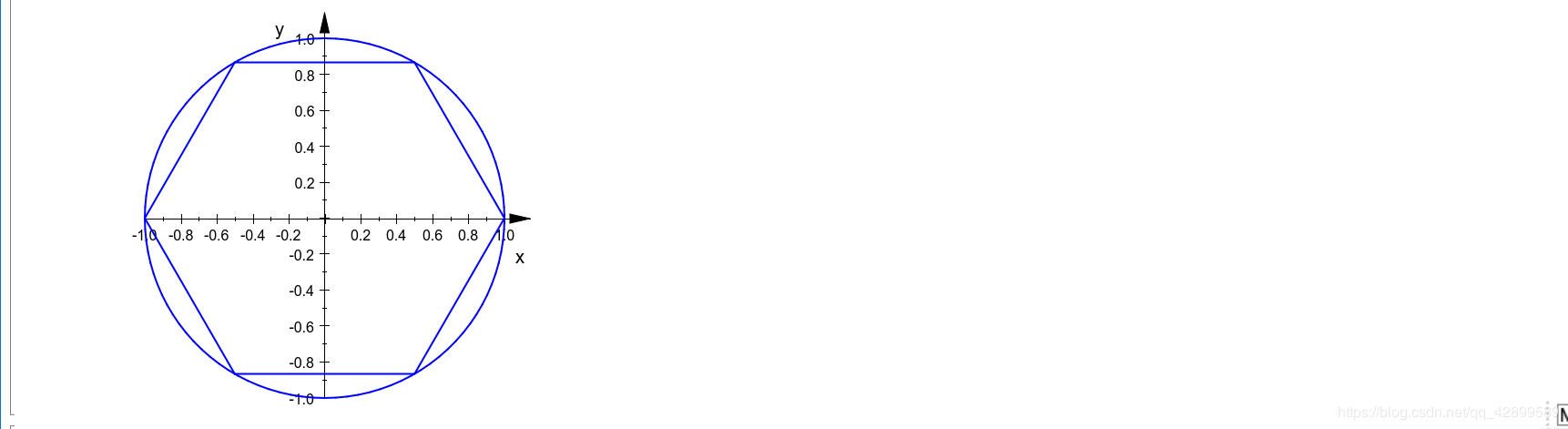4.绘制矩形
plot::Rectangle(xmin…xmax,ymin…ymax,<动画参数>,<属性>)
xmin…xmax 矩形的左右边界
ymin…ymax 矩形的上下边界

LineVisible：线是否显示
Filled：内部是否填充

## 七、计算极限

limit(f,x=x0) 计算双侧极限
limit(f,x=x0,Left) 计算左侧极限
limit(f,x=x0,Right) 计算右侧极限

f：函数或数列表达式
x=x0 : 被求极限的位置，x0也可以换成也可以是infinity或-infinity

``````limit(1/x,x=infinity,Left);
limit(1/x,x=-infinity,Right);//结果都是0
``````

1.多项式除法运算
divide(P,Q,[x])

P:分子的多项式表达式
Q:分母的多项式表达式
[x]:多项式的变量

2.解方程或不等式
solve(f,x)
solve(f,x=a…b) 返回函数在[a,b]之间的零点

`````` S:=solve(x^2=2,x);
``````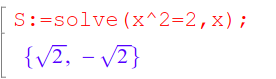``````float(S);
``````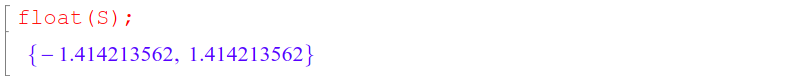系统默认是保留十位有效数字的，如果想保留更多位：

``````DIGITS:20:float(solve(x^2=2,x));
``````
``````solve(x-2>2,x);//解不等式，结果是4到正无穷
``````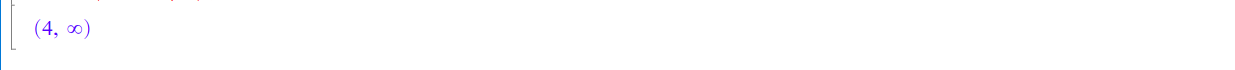3.其他命令
linsolve：求解线性方程组
RootOf：求解多项式的根
testeq：检验两个表达式是否相等

o

### osc_wws45aot

06/11
0
0

机器之心报道 　　参与：泽南、杜伟 　　 　　 一个商业数学软件居然也能被美国人禁用。 　　哈工大、哈工程的老师和学生们最近无法使用 MATLAB 了，这一消息迅速成为了人们关注的热点。...

osc_7co5s4uj
06/12
28
0

机器之心报道 　　参与：泽南、杜伟 　　 　　 一个商业数学软件居然也能被美国人禁用。 　　哈工大、哈工程的老师和学生们最近无法使用 MATLAB 了，这一消息迅速成为了人们关注的热点。...

06/11
0
0

06/15
0
0

3Ｄ视觉工坊
06/13
0
0

dict.items（）和dict.iteritems（）有什么区别？

20
0
R中“ =”和“ <-”赋值运算符有什么区别？

fyin1314

20
0

javail

15
0

7
0

10
0